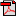# Lesson 1: Getting Ready to Multiply

## Getting Started

### Questions to Explore

• What are some strategies we can use to multiply and divide?

### Facts and Definitions

• Array: an ordered arrangement of objects in equal rows and columns

### Skills

• Skip count
• Use arrays to create addition sentences
• Identify equal groups

### Materials

• The Grapes of Math by Greg Tang
• abacus (kit)
• base-10 block cards (kit)
• colored pencils
• egg carton
• fine point dry-erase markers (kit)
• Interactive Notebook
• whiteboard (kit)

### Introduction

Materials: abacus (kit), base-10 block cards (kit), fine point dry-erase markers (kit), Interactive Notebook, whiteboard (kit)
This unit introduces your child to multiplication and division and focuses on the factors 2, 3, 4, 5, and 10. Your child will explore the relationship between multiplication and division and will begin to memorize his multiplication facts. In addition, he will complete occasional review activities focused on basic skills such as adding and subtracting to 1000, telling time, and counting money. While this unit introduces both multiplication and division, the main focus is on multiplication. Division will be addressed more fully during Unit 4.

Write the following number sentences vertically (top to bottom) on the whiteboard and ask your child to read them aloud:
• 55 + 30
• 74 − 50
• 350 + 70
• 420 − 40
For example, he should say "fifty-five plus thirty" for the first one. Now, ask him to explain what he knows about the numbers in each number sentence. He might tell how many digits each number has and which digits are in which places in each number. For example, for 350, he might say that the number has three digits and that the 3 is in the hundreds place, the 5 is in the tens place, and the 0 is in the ones place. Prompt him to provide this information as needed.
Once your child has had a chance to discuss each of the number sentences, give him time to find the sums and differences. (85, 24, 420, 380) Allow him to use materials such as the base-10 blocks card or the abacus as needed, and encourage him to use the math strategies that he already knows to find the answers. For example, he can add and subtract the ones places first and then use carrying or borrowing as needed. Give him time to correct any mistakes. For a review of some math strategies from previous units, see the "Math Strategies Review" sheets, and review the strategies with your child as needed. Store these sheets in your child's Interactive Notebook for future reference as needed.

Now, say, "You've already worked with addition and subtraction, and you know how to add and subtract numbers all the way up to 1000. During this unit, you're going to learn about two more mathematical operations that we use in our daily lives. Before you get into these operations, though, you'll review some skills that will help you better understand them."Student Activity PageStudent Activity PageStudent Activity Page